Courses

# 17Th July 2016- XII

## 40 Questions MCQ Test | 17Th July 2016- XII

Description
This mock test of 17Th July 2016- XII for Class 12 helps you for every Class 12 entrance exam. This contains 40 Multiple Choice Questions for Class 12 17Th July 2016- XII (mcq) to study with solutions a complete question bank. The solved questions answers in this 17Th July 2016- XII quiz give you a good mix of easy questions and tough questions. Class 12 students definitely take this 17Th July 2016- XII exercise for a better result in the exam. You can find other 17Th July 2016- XII extra questions, long questions & short questions for Class 12 on EduRev as well by searching above.
QUESTION: 1

Solution:
QUESTION: 2

Solution:
QUESTION: 3

### The instrument for the accurate measurement of the e.m.f of a cell is

Solution:
QUESTION: 4

Potentiometer measures the potential difference more accurately than a voltmeter, because

Solution:
QUESTION: 5

In a potentiometer experiment, for measuring internal resistance of a cell, the balance point has been obtained on the fourth wire. The balance point can be shifted to fifth wire by

Solution:
QUESTION: 6

The sensitivity of the potentiometer can be increased by

Solution:
QUESTION: 7

Two resistances are connected in the two gaps of a meter bridge. The balance points is 20 cm from the zero end. When a resistance of 15 ohm is connected in series with the smaller of the two resistances, the null point shifts to 40 cm. The smaller of the two resistances has the value (in ohm)

Solution:
QUESTION: 8

Kirchhoff’s second law is a consequence of

Solution:
QUESTION: 9

In a meter bridge, when galvanometer & cell positions are interchanged at balanced point

Solution:
QUESTION: 10

Figure shows current in a part of an electrical circuit. Then current I is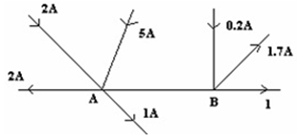Solution:
QUESTION: 11

The figure shows an unbalanced Wheatstone bridge. To balance the bridge: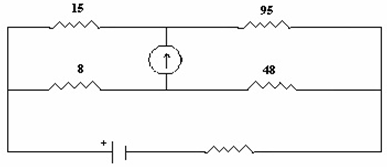Solution:
QUESTION: 12

In a meter bridge experiment a balance point is obtained at a distance of 60 cm from the left end when unknown resistance R is in a left gap and 8 ohms resistor is connected in the right gap. When the position of R and 8 ohm resistor is interchanged the balance point will be at distance of

Solution:
QUESTION: 13

The Wheatstone bridge is balanced for four resistors R1,R2,R3 and R4 with a cell of emf 1.46 V. The cell is now replaced by another cell of emf 1.08 V. To obtain the balance again

Solution:
QUESTION: 14

Meter Bridge is based on the principle of

Solution:
QUESTION: 15

In the circuit shown, the cell has e m f = 10 V and internal resistance = 1Ω1Ω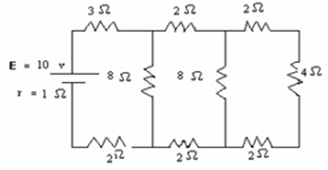Solution:
QUESTION: 16

Electromotive force is

Solution:
QUESTION: 17

Current density of a conductor is

Solution:
QUESTION: 18

Mobility is defined as

Solution:
QUESTION: 19

The wire of the potentiometer has resistance 4 ohms and length 1 m. It is connected to a cell of e.m.f. 2 volts and internal resistance 1 ohm, the potential gradient in the potentiometer wire is:

Solution:
QUESTION: 20

The wire of the potentiometer has resistance 4 ohms and length 1 m. It is connected to a cell of e.m.f. 2 volts and internal resistance 1 ohm, if a cell of e.m.f. 1.2 volt is balanced by it, the balancing length will be

Solution:
QUESTION: 21

A potentiometer has a uniform wire of length 10m and resistance . The potentiometer is connected to an external battery of emf of 10V and negligible internal resistance and a resistance of in series. The potential gradient along the were is:

Solution:
QUESTION: 22

In a Wheatstone’s bridge, P = 9 ohms, Q = 11 ohms, R = 4 ohms and S = 6 ohms. How much resistance must be put in series to the resistance S to balance the bridge?

Solution:
QUESTION: 23

According to Ohm's law

Solution:
QUESTION: 24

Drift is the random motion of the charged particles within a conductor

Solution:
QUESTION: 25

According to Kirchhoff’s Junction Rule

Solution:
QUESTION: 26

Direction of the conventional current

Solution:
QUESTION: 27

According to Kirchhoff’s Loop Rule

Solution:
QUESTION: 28

Potentiometer is

Solution:
QUESTION: 29

In the circuit shown in fig E, F and G and H are cells of emf 2 V, 1 V, 3 V and 1V and their internal resistances are 2Ω, 1 Ω, 3 Ω and 1 Ω respectively. The potential difference between B and D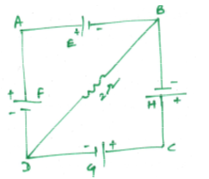Solution:
QUESTION: 30

Constantan or manganin are used for making standard resistors

Solution:
QUESTION: 31

Potential difference across terminals of a cell were measured (in volt) against different currents( in ampere) flowing through the cell. A graph was drawn which was a straight line ABC, as shown in below. Determine from the graph. Internal resistance of the cell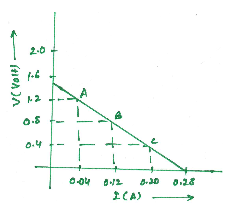Solution:
QUESTION: 32

The resistance of a semi-conductors

Solution:
QUESTION: 33

The terminal voltage across a battery of emf E can be

Solution:
QUESTION: 34

Two wires of the same material having radii in the ratio 1:2, carry currents in the ratio 4 : 1. The ratio of drift velocities of electrons in them is:

Solution:
QUESTION: 35

In the potentiometer circuit shown the balance (null) point is at X. Where the balance point will be shifted when Resistance S increases keeping all other parameters contanst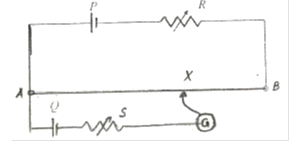Solution:
QUESTION: 36

Two wires of equal lengths one of copper and the other of manganin have the same resistance. Which wire will be thicker?

Solution:
QUESTION: 37

The Wheatstone bridge of fig is showing no deflection in the galvanometer joined between the point B and D. compute the value of R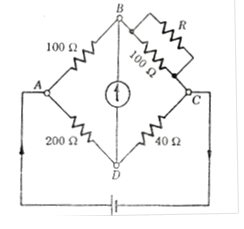Solution:
QUESTION: 38

A copper wire has a resistance of 10 Ω and an area of cross section 1mm2. A potential difference of 10 V exists across the wire. The drift speed of electrons if the number of electrons per cubic meter in copper is 8 × 1028 electrons is

Solution:
QUESTION: 39

A cylindrical wire is stretched to increase its length by 10%. Calculate the percentage increase its length by 10%. Calculate the percentage increase in resistance

Solution:
QUESTION: 40

A current of 2 mA is passed through a color coded carbon resistor with first, second and third rings of yellow, green and orange colors. the voltage drop across the resistor is

Solution: